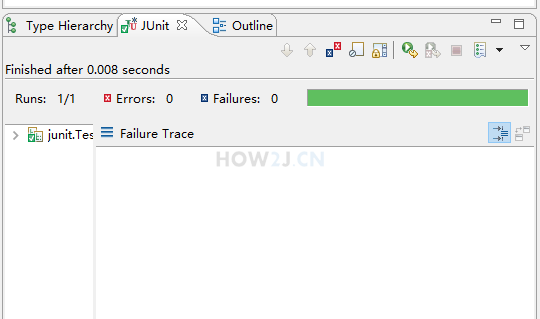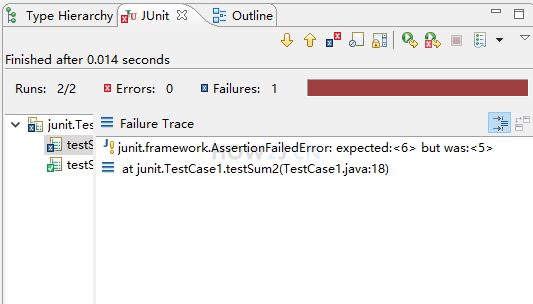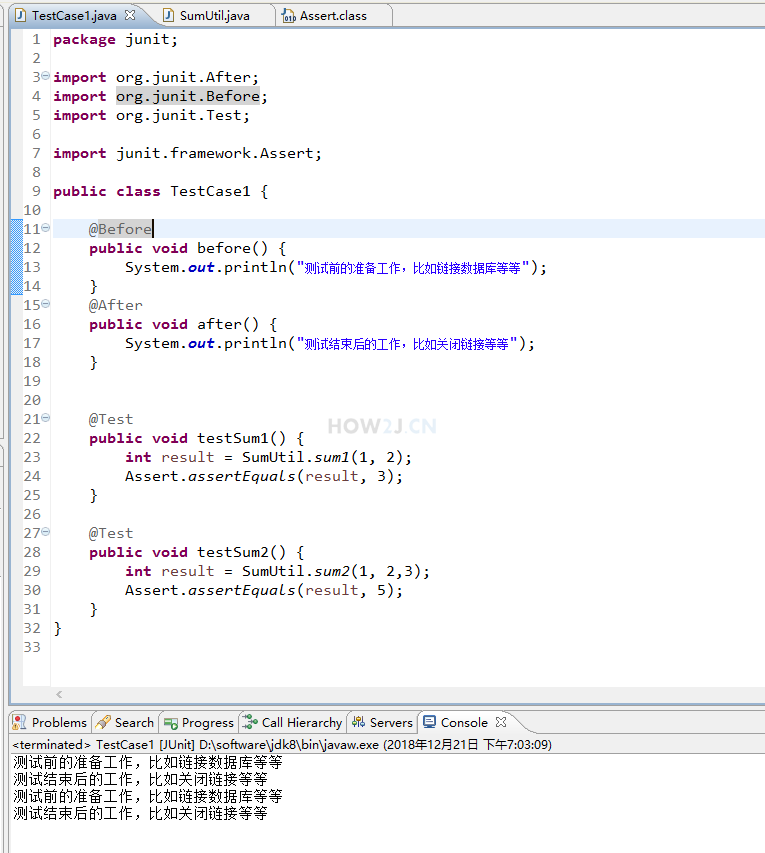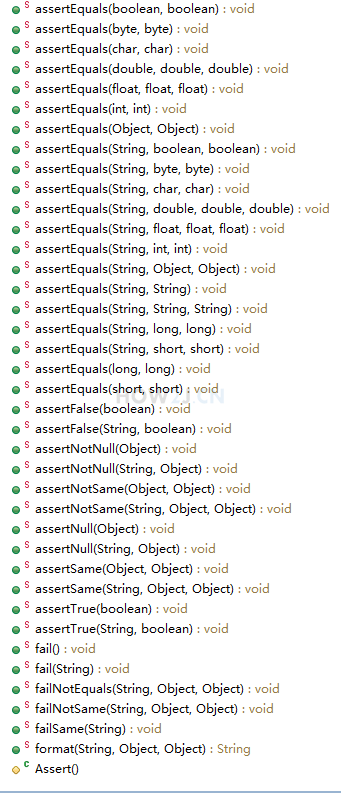junit-4.9.jar 243k
junit.rar 211k

## 用 main 进行测试

package junit; public class SumUtil { public static void main(String[] args) { int result = sum1(1,2); System.out.println(result); } public static int sum1(int i, int j) { return i+j; } }
```package junit;

public class SumUtil {

public static void main(String[] args) {
int result = sum1(1,2);
System.out.println(result);
}

public static int sum1(int i, int j) {
return i+j;
}

}
```

## main 的问题

1. 要在原来测试的基础上修改，可能破坏原来的测试逻辑
2. 测试成功了，还是i测试失败了，都不知道呀。。。只能通过肉眼进行观察，如果测试的量很大，是很难看的过来滴
package junit; public class SumUtil { public static void main(String[] args) { int result = sum1(1,2); System.out.println(result); int result2 = sum2(1,2,3); System.out.println(result2); } public static int sum1(int i, int j) { return i+j; } public static int sum2(int i, int j, int k) { return i+j+k; } }
```package junit;

public class SumUtil {

public static void main(String[] args) {
int result = sum1(1,2);
System.out.println(result);
int result2 = sum2(1,2,3);
System.out.println(result2);
}

public static int sum1(int i, int j) {
return i+j;
}

public static int sum2(int i, int j, int k) {
return i+j+k;
}

}
```

## junit

@Test
public void testSum1() {
int result = SumUtil.sum1(1, 2);
Assert.assertEquals(result, 3);
}

Assert.assertEquals(result, 3); 表示对 result 数值的期待是 3，如果是其他数值，就无法通过测试。
package junit; import org.junit.Test; import junit.framework.Assert; public class TestCase1 { @Test public void testSum1() { int result = SumUtil.sum1(1, 2); Assert.assertEquals(result, 3); } }
```package junit;

import org.junit.Test;

import junit.framework.Assert;

public class TestCase1 {

@Test
public void testSum1() {
int result = SumUtil.sum1(1, 2);
Assert.assertEquals(result, 3);
}

}
```

## 运行方式## 运行成功## 新增加一个测试方法

Assert.assertEquals(result, 5);

package junit; import org.junit.Test; import junit.framework.Assert; public class TestCase1 { @Test public void testSum1() { int result = SumUtil.sum1(1, 2); Assert.assertEquals(result, 3); } @Test public void testSum2() { int result = SumUtil.sum2(1, 2,3); Assert.assertEquals(result, 5); } }
```package junit;

import org.junit.Test;

import junit.framework.Assert;

public class TestCase1 {

@Test
public void testSum1() {
int result = SumUtil.sum1(1, 2);
Assert.assertEquals(result, 3);
}

@Test
public void testSum2() {
int result = SumUtil.sum2(1, 2,3);
Assert.assertEquals(result, 5);
}
}
```

## 运行失败## junit的好处

1. 新增加的测试，对原来的测试没有影响
2. 如果测试失败了，会立即得到通知

## before & after

@Before @After 也是常见的测试框架注解，分别用来在测试开始之前做的事情，和结束之后做的事情。package junit; import org.junit.After; import org.junit.Before; import org.junit.Test; import junit.framework.Assert; public class TestCase1 { @Before public void before() { System.out.println("测试前的准备工作，比如链接数据库等等"); } @After public void after() { System.out.println("测试结束后的工作，比如关闭链接等等"); } @Test public void testSum1() { int result = SumUtil.sum1(1, 2); Assert.assertEquals(result, 3); } @Test public void testSum2() { int result = SumUtil.sum2(1, 2,3); Assert.assertEquals(result, 5); } }

## AssertHOW2J公众号，关注后实时获知最新的教程和优惠活动，谢谢。2021-08-10 提示：如果使用IDEA，并且项目为Maven工程的话，测试的代码需要放在创建maven时自动生成的test包下
 橘子西瓜 关于 JAVA 应用-junit-入门教程 的提问 提示：如果使用IDEA，并且项目为Maven工程的话，测试的代码需要放在创建maven时自动生成的test包下

2020-10-27 eclipse 右键没有Run as junit
 Ocarina 关于 JAVA 应用-junit-入门教程 的提问 导入了jar包但是右键死活没有junit，百度也没能解决问题，有遇到同样问题的朋友吗？

3 个答案

M_GX

`方法之前加个@Test就能看到了，这个是注解，只有加了这个才能进行测试`

Javeson

BianJiang

2020-03-09 idea找不到runas选项
2020-02-28 工作中的单元测试
2020-01-04 关于 @before，@after 和 @beforeclass ，@afterclas